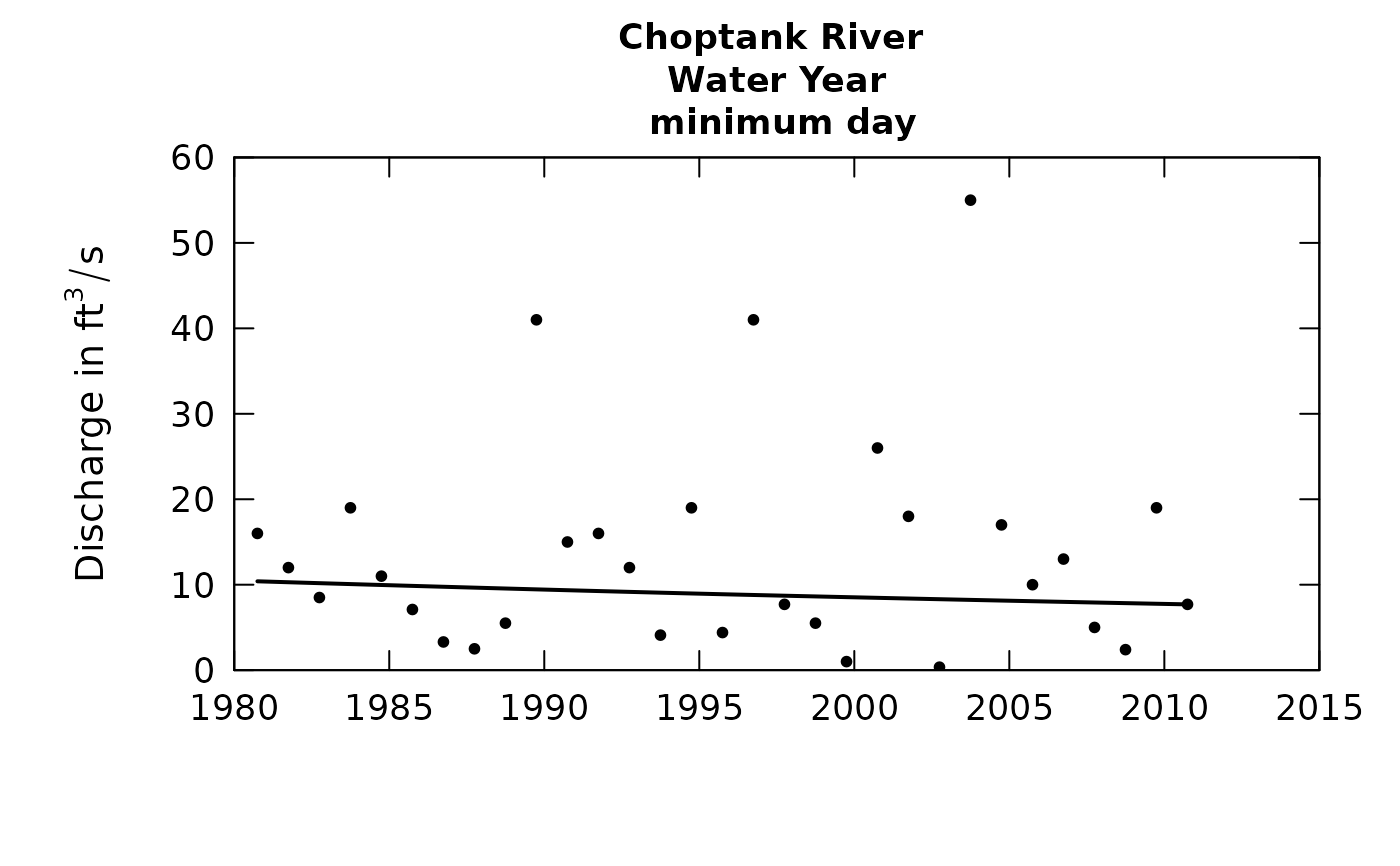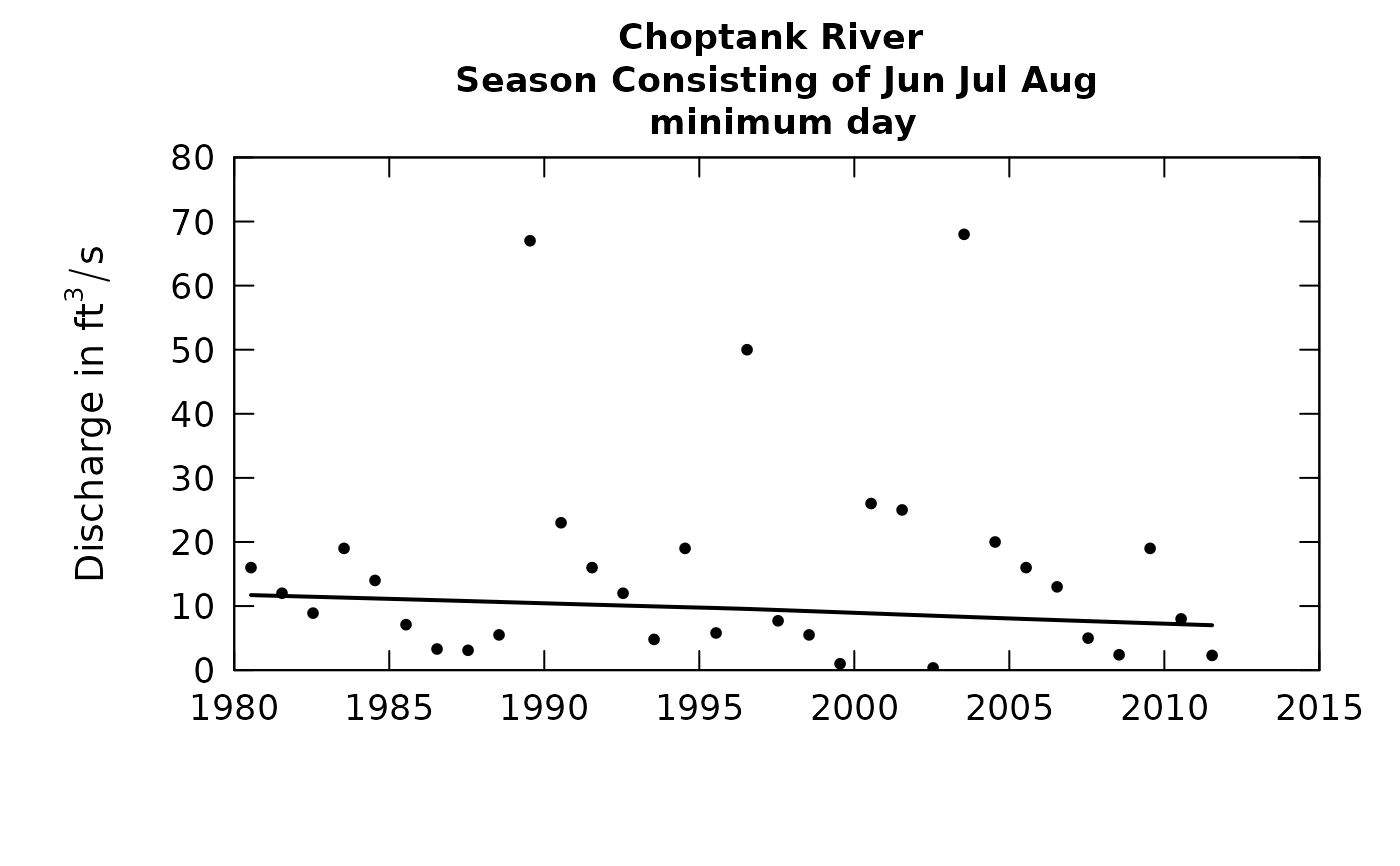A part of the flowHistory system. The index of the flow statistics is istat. These statistics are: (1) 1-day minimum, (2) 7-day minimum, (3) 30-day minimum, (4) median (5) mean, (6) 30-day maximum, (7) 7-day maximum, and (8) 1-day maximum

Although there are a lot of optional arguments to this function, most are set to a logical default.

Data come from named list, which contains a Daily dataframe with the daily flow data, and an INFO dataframe with metadata.

## Usage

plotFlowSingle(eList, istat, yearStart = NA, yearEnd = NA, qMax = NA,
printTitle = TRUE, tinyPlot = FALSE, customPar = FALSE,
runoff = FALSE, qUnit = 1, printStaName = TRUE, printPA = TRUE,
usgsStyle = FALSE, printIstat = TRUE, cex = 0.8, cex.axis = 1.1,
cex.main = 1.1, lwd = 2, col = "black", ...)

## Arguments

eList

named list with at least the Daily and INFO dataframes

istat

A numeric value for the flow statistic to be graphed (possible values are 1 through 8)

yearStart

A numeric value for year in which the graph should start, default is NA, which indicates that the graph should start with first annual value

yearEnd

A numeric value for year in which the graph should end, default is NA, which indicates that the graph should end with last annual value

qMax

A numeric value for the maximum value to be used for y-axis of graph, default is NA means that graph is self-scaling

printTitle

logical variable, if TRUE title is printed, if FALSE title is not printed, default is TRUE

tinyPlot

logical variable, if TRUE plot is designed to be plotted small, as a part of a multipart figure, default is FALSE

customPar

logical defaults to FALSE. If TRUE, par() should be set by user before calling this function (for example, adjusting margins with par(mar=c(5,5,5,5))). If customPar FALSE, EGRET chooses the best margins depending on tinyPlot.

runoff

logical variable, if TRUE the streamflow data are converted to runoff values in mm/day

qUnit

object of qUnit class printqUnitCheatSheet, or numeric represented the short code, or character representing the descriptive name.

printStaName

logical variable, if TRUE station name is printed in title, if FALSE not printed, default is TRUE

printPA

logical variable, if TRUE Period of Analysis information is printed in title, if FALSE not printed, default is TRUE

usgsStyle

logical option to use USGS style guidelines. Setting this option to TRUE does NOT guarantee USGS compliance. It will only change automatically generated labels.

printIstat

logical variable, if TRUE print the statistic name is printed in title, if FALSE not printed, default is TRUE

cex

numerical value giving the amount by which plotting symbols should be magnified

cex.axis

magnification to be used for axis annotation relative to the current setting of cex

cex.main

magnification to be used for main titles relative to the current setting of cex

lwd

number line width

col

color of points on plot, see ?par 'Color Specification'

...

arbitrary graphical parameters that will be passed to genericEGRETDotPlot function (see ?par for options)

## Details

The curve plotted on the graph is a loess smooth of the data. This smooth is computed on the logs of the data and then transformed back to plot. The width of the smoothing window is 20 years on either side of the year being plotted However, the window width can be adjusted using setPA function.

makeAnnualSeries, genericEGRETDotPlot

## Examples

eList <- Choptank_eList
# Water year:
plotFlowSingle(eList, 1)# Graphs consisting of Jun-Aug
eList <- setPA(eList, paStart=6,paLong=3)
plotFlowSingle(eList, 1)